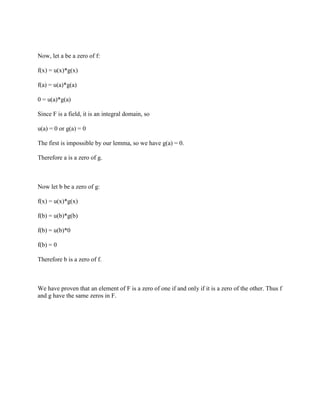Diese Präsentation wurde erfolgreich gemeldet.

# Please cite all of the properties- theorems- corollaries and definitio.docx

Anzeige
Anzeige
Anzeige
Anzeige
Anzeige
Anzeige
Anzeige
Anzeige
Anzeige
Anzeige
Anzeige×

1 von 3 Anzeige

# Please cite all of the properties- theorems- corollaries and definitio.docx

Please cite all of the properties, theorems, corollaries and definitions you use.

Let F be a field. If f(x) and g(x) are associates in F[x], prove that the two polynomials have the same roots in F.
Solution
For simplicity, we call the multiplicative unit of F 1, and we identify the multiplicative unit of F[x] as 1(x)

Since f(x) and g(x) are associates in F[x], there exists a unit u(x) in F[x] such that:

f(x) = u(x)*g(x)

First a lemma: u(x) has no zeros in F.
Proof: u(x) a unit implies there exists an element v(x) in F[x] such that u(x)*v(x) = 1(x)
If s were a zero of u(x), then plugging into our uv=1 equation:
u(x)*v(x) = 1(x)
u(s)*v(s) = 1 [Note: 1(x) = 1 for all values of x]
0*v(s) = 1
0 = 1
Contradiction. Thus u has no zeros.

Now, let a be a zero of f:
f(x) = u(x)*g(x)
f(a) = u(a)*g(a)
0 = u(a)*g(a)
Since F is a field, it is an integral domain, so
u(a) = 0 or g(a) = 0
The first is impossible by our lemma, so we have g(a) = 0.
Therefore a is a zero of g.

Now let b be a zero of g:
f(x) = u(x)*g(x)
f(b) = u(b)*g(b)
f(b) = u(b)*0
f(b) = 0
Therefore b is a zero of f.

We have proven that an element of F is a zero of one if and only if it is a zero of the other. Thus f and g have the same zeros in F.

.

Please cite all of the properties, theorems, corollaries and definitions you use.

Let F be a field. If f(x) and g(x) are associates in F[x], prove that the two polynomials have the same roots in F.
Solution
For simplicity, we call the multiplicative unit of F 1, and we identify the multiplicative unit of F[x] as 1(x)

Since f(x) and g(x) are associates in F[x], there exists a unit u(x) in F[x] such that:

f(x) = u(x)*g(x)

First a lemma: u(x) has no zeros in F.
Proof: u(x) a unit implies there exists an element v(x) in F[x] such that u(x)*v(x) = 1(x)
If s were a zero of u(x), then plugging into our uv=1 equation:
u(x)*v(x) = 1(x)
u(s)*v(s) = 1 [Note: 1(x) = 1 for all values of x]
0*v(s) = 1
0 = 1
Contradiction. Thus u has no zeros.

Now, let a be a zero of f:
f(x) = u(x)*g(x)
f(a) = u(a)*g(a)
0 = u(a)*g(a)
Since F is a field, it is an integral domain, so
u(a) = 0 or g(a) = 0
The first is impossible by our lemma, so we have g(a) = 0.
Therefore a is a zero of g.

Now let b be a zero of g:
f(x) = u(x)*g(x)
f(b) = u(b)*g(b)
f(b) = u(b)*0
f(b) = 0
Therefore b is a zero of f.

We have proven that an element of F is a zero of one if and only if it is a zero of the other. Thus f and g have the same zeros in F.

.

Anzeige
Anzeige

### Please cite all of the properties- theorems- corollaries and definitio.docx

1. 1. Please cite all of the properties, theorems, corollaries and definitions you use. Let F be a field. If f(x) and g(x) are associates in F[x], prove that the two polynomials have the same roots in F. Solution For simplicity, we call the multiplicative unit of F 1, and we identify the multiplicative unit of F[x] as 1(x) Since f(x) and g(x) are associates in F[x], there exists a unit u(x) in F[x] such that: f(x) = u(x)*g(x) First a lemma: u(x) has no zeros in F. Proof: u(x) a unit implies there exists an element v(x) in F[x] such that u(x)*v(x) = 1(x) If s were a zero of u(x), then plugging into our uv=1 equation: u(x)*v(x) = 1(x) u(s)*v(s) = 1 [Note: 1(x) = 1 for all values of x] 0*v(s) = 1 0 = 1 Contradiction. Thus u has no zeros.
2. 2. Now, let a be a zero of f: f(x) = u(x)*g(x) f(a) = u(a)*g(a) 0 = u(a)*g(a) Since F is a field, it is an integral domain, so u(a) = 0 or g(a) = 0 The first is impossible by our lemma, so we have g(a) = 0. Therefore a is a zero of g. Now let b be a zero of g: f(x) = u(x)*g(x) f(b) = u(b)*g(b) f(b) = u(b)*0 f(b) = 0 Therefore b is a zero of f. We have proven that an element of F is a zero of one if and only if it is a zero of the other. Thus f and g have the same zeros in F.